# SVG Coordinate Transformations

This topic covers SVG coordinate transformations that are associated with the `getScreenCTM()` method.

The subject of SVG coordinates and transformations is large. You can find basic information about this subject from the W3C SVG specification – specifically Coordinate Systems, Transformations and Units.

This topic covers a particularly pernicious issue associated with SVG coordinates – the mapping of a point in screen coordinates (technically, the initial viewport coordinate system) to the coordinate system associated with any given SVG element (technically, the current user coordinate system). For example, consider an SVG circle using a standard Cartesian coordinate system:Figure 1

In this example, we are interested in determining the position of the mouse relative to the circle’s coordinate system. Thus, we must map the mouse’s screen coordinates to the circle’s Cartesian coordinates as suggested by the following figure: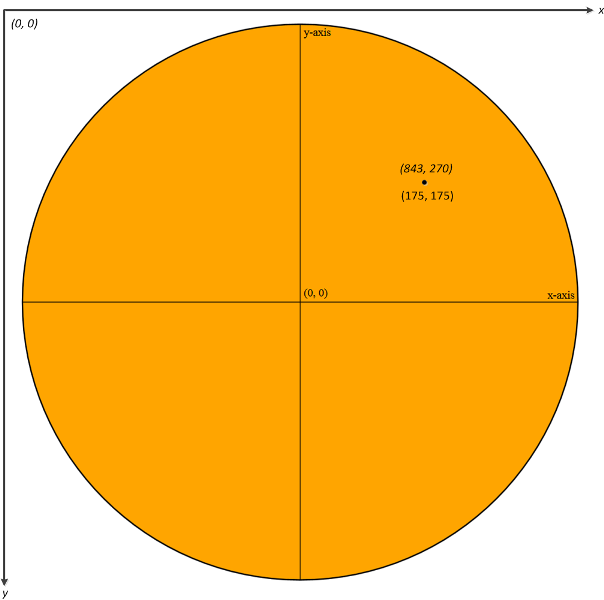Figure 2

In Figure 2, the mouse has a screen coordinate of (843, 270). When this point is mapped to the circle’s coordinate system, the mouse coordinate becomes (175, 175).

Note

If you’re not familiar with matrices, matrix multiplication, or matrix inversion, consider reviewing one or more of the following before proceeding:

To describe the transformation in Figure 2 mathematically, it is helpful to start with a few definitions. From the W3C SVG specification, we have:

Transformation matrices define the mathematical mapping from one coordinate system into another. The current transformation matrix (CTM) defines the mapping from the user coordinate system into the viewport coordinate system using a 3x3 CTM matrix via the following equation: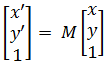where,

• M is the current transformation matrix (CTM).
•is a homogenous vector that represents a point (x, y) in the user coordinate system. In Figure 2, this vector is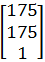, which is equivalent to "circle point" (175, 175). Be aware that the "1" insimply allows for matrix arithmetic and can essentially be ignored.
•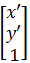is a homogenous vector that represents a point (x’, y’) in the viewport coordinate system. In Figure 2, this vector is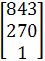, which is equivalent to "screen point" (843, 270).

Fortunately, the CTM can be acquired in JavaScript by invoking the `getScreenCTM()` method. For example, if the circle in Figure 2 is named `svgCircle`, then `var M = svgCircle.getScreenCTM()` would return the appropriate matrix M to convert a circle point to a screen point using the previous equation. That is, if the current transformation matrix M for Figure 2 is:Then we can map circle coordinate (175, 175) to screen coordinate (843, 270) as follows:Which, after rounding, produces screen coordinate (843, 270).

The previous example shows how to transform a circle coordinate to a screen coordinate, but what about the inverse? That is, given a screen coordinate, what is the corresponding circle coordinate? To answer that question, we must solvefor. This can be accomplished as follows:where,

• M⁻¹ is the inverse of the CTM matrix, which is approximately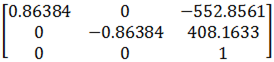for the above example. Because all allowed SVG coordinate transformations map a 2D space to another 2D space, M will always be invertible.
• I represents the 3x3 identity matrix (recall that M⁻¹ = I).

Thus using our derived equation, we can map screen coordinate (843, 270) to its corresponding circle coordinate as follows: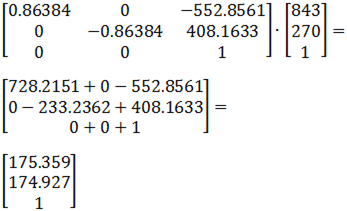Which, after rounding, produces circle coordinate (175, 175).

With this mathematical understanding in place, implementingin JavaScript is relatively straightforward:

``````function coordinateTransform(screenPoint, someSvgObject)
{
var CTM = someSvgObject.getScreenCTM();
return screenPoint.matrixTransform( CTM.inverse() );
}
``````

This function, given a screen point (of type `SVGPoint`) and some SVG object such as a circle, will map the screen point to the coordinate system associated with `someSvgObject`. If `someSvgObject` represents the circle of Figure 2, then:

• `var CTM = someSvgObject.getScreenCTM()` gets the CTM associated with the current screen and circle size.
• `CTM.inverse()` inverts the CTM matrix, giving us M⁻¹ in the prior equation.
• `screenPoint.matrixTransform( CTM.inverse() )` multiplies M⁻¹ and the given screen coordinate(that is, `screenPoint`) to produce the required circle coordinate.

To view the full sample associated with Figure 2, click Liquid SVG. To view the source code associated with this sample, use the view source feature of your browser. For example, in Windows Internet Explorer, right-click the webpage and choose View source. Make sure you have the source code available while reading the remainder of this document.

Be aware that as you change the size of the browser window, the circle size adjusts accordingly. This liquid layout is the result of the following percent-based values:

``````html {
margin: 0;
height: 100%; /* Required for liquidity. */
}

body {
margin: 0;
height: 100%; /* Required for liquidity. */
}
``````

and

``````<!-- width="100%" and height="98%" required for liquidity. -->
<svg id="svgElement" width="100%" height="98%" viewbox="0 0 800 800">
``````

Moving to a different aspect of the code, the `svgCircle` element, as well as its sibling elements, are given a standard Cartesian coordinate system via the following two `<g>` element transforms:

``````<g transform="translate(400, 400) scale(1, -1)">
``````

That is, `translate(400, 400)` moves the origin of the viewport’s coordinate system to the point (400, 400) relative to the 800x800 view box imposed on the `svg` element via `viewbox="0 0 800 800"`. The `scale(1, -1)` transform then scales the user coordinate system’s x-axis by 1 (no change) and the y-axis by -1, which has the effect of flipping the y-axis about the x-axis. The result is a standard Cartesian coordinate system (whose origin occurs in the middle of the 800x800 viewport).

The remaining (minor) details of the sample are covered via the copious comments sprinkled throughout Liquid SVG. To see how this technique is used in an SVG-based video game, see Example 6 of Advanced SVG Animation.

HTML5 Graphics

How to Add SVG to a Webpage